Next: Zero Crossings of the Laplacian of a Up: Edges Previous: Edges

## Early Uses of the First Image Brightness Derivative

Some of the earliest methods of finding edges in images used small convolution masks to approximate the first derivative of the image brightness function, thus enhancing edges. Roberts (see ) used masks of size two pixels by two pixels to find orthogonal derivatives;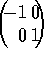and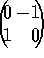. Prewitt (see ) used masks of size three pixels by three pixels in a similar way;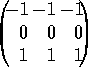and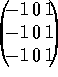. This is preferred to Roberts' approach because of the slightly greater amount of noise suppression, the fact that the gradient image is not shifted by half a pixel in both directions, and because extension to higher image dimensions is not possible with the Roberts operator. Sobel (see ) used slightly different masks to Prewitt, in that ``Gaussian averaging'' is performed in one direction, and differentiation is performed in the other;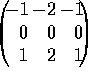and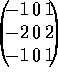. In  the Sobel filter is analyzed in terms of separable two pixel by one pixel components, and the extension to larger filters and more dimensions is made. The image gradient magnitude for all three of these methods is found by taking the square root of the sum of the squares of the outputs from the two orthogonal masks. Likewise, the gradient direction can be found from the ratio of the outputs of the masks.

Other small mask operators have been suggested, such as ``compass'' operators, which have more than two masks, oriented in several directions. In  the responses to gradient magnitude of several small gradient masks are analyzed. It is shown that the choice of mask should depend on the type of image noise present. It is also shown that the isotropy of response does not depend much on the choice of mask.Next: Zero Crossings of the Laplacian of a Up: Edges Previous: Edges

© 1997 Stephen M Smith. LaTeX2HTML conversion by Steve Smith (steve@fmrib.ox.ac.uk)